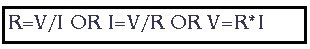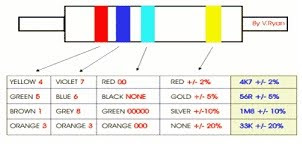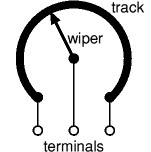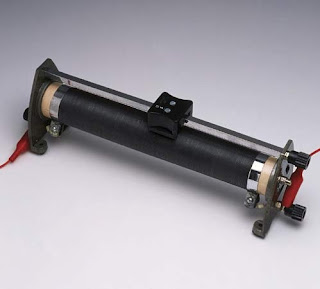## Breaking

### BASIC ELECTRONIC COMPONENTS (RESISTOR)

1. Resistors:
1.1. Usefulness:
-Resistors determine the flow of current in an electrical circuit. Where there is high resistance then the flow of current is small, where the resistance is low the flow of current is large.

Fig (1) charge flow before and after passing the resistorResistance, voltage and current are connected in an electrical circuit by Ohm’s Law.Resistors are used for regulating current and they resist the current flow and the extent to which they do this is measured in ohms .Resistors are found in almost every electronic circuit.
1.2. How to calculate the resistance:
Resistors are too small to have numbers printed on them and so they are marked with a number of colored bandsFig (2) color code
Each color stands for a number .Three color bands show the resistors value in ohms and the fourth shows tolerance.
- Resistors can never be made to a precise value and the tolerance band (the fourth band) tells us, using a percentage, how close the resistor is to its coded value.
1.3. Resistor configurations:
1.3.1.Resistors in series:
When resistors are connected in series, their values are added together:
Rtotal=R1+R2
1.3.2.Resistors in parallel:
When resistors are connected in parallel, their total resistance is given as:1/Rtotal = 1/R1 + 1/R2

1.4.Resistor kinds:
1.4.1.Constant resistor:Is the ordinary resistor as discussed above
Fig (3) Constant resistor
1.4.2. Variable resistors:Variable resistors consist of a resistance track with connections at both ends and a wiper which moves along the track as you turn the spindle. The track may be made from carbon and cermets ceramic .The track is usually rotary but straight track versions, usually called sliders, are also available variable resitpoor image.
Fig(3) Variable resistor with rotary trackFig (4) Variable resistor with steady track
1.4.3. LDR:
-A light dependant resistor, LDR, has a high resistance when it's dark, and a low resistance when it's light.
-They are made out of silicon.
-A thermistor looks like a LDR but it doesn't respond to light, but to heat.
- It can be used to measure temperatures or in thermostats.
-Often semi-conducting materials are used that get a lower resistance when de temperature rises, this is called negative temperature coefficient (NTC).
-All normal resistors have a small positive temperature coefficient (resistance becomes higher when the temperature becomes higher).### BASIC ELECTRONIC COMPONENTS (RESISTOR)

1. Resistors:
1.1. Usefulness:
-Resistors determine the flow of current in an electrical circuit. Where there is high resistance then the flow of current is small, where the resistance is low the flow of current is large.

Fig (1) charge flow before and after passing the resistorResistance, voltage and current are connected in an electrical circuit by Ohm’s Law.Resistors are used for regulating current and they resist the current flow and the extent to which they do this is measured in ohms .Resistors are found in almost every electronic circuit.
1.2. How to calculate the resistance:
Resistors are too small to have numbers printed on them and so they are marked with a number of colored bandsFig (2) color code
Each color stands for a number .Three color bands show the resistors value in ohms and the fourth shows tolerance.
- Resistors can never be made to a precise value and the tolerance band (the fourth band) tells us, using a percentage, how close the resistor is to its coded value.
1.3. Resistor configurations:
1.3.1.Resistors in series:
When resistors are connected in series, their values are added together:
Rtotal=R1+R2
1.3.2.Resistors in parallel:
When resistors are connected in parallel, their total resistance is given as:1/Rtotal = 1/R1 + 1/R2

1.4.Resistor kinds:
1.4.1.Constant resistor:Is the ordinary resistor as discussed above
Fig (3) Constant resistor
1.4.2. Variable resistors:Variable resistors consist of a resistance track with connections at both ends and a wiper which moves along the track as you turn the spindle. The track may be made from carbon and cermets ceramic .The track is usually rotary but straight track versions, usually called sliders, are also available variable resitpoor image.
Fig(3) Variable resistor with rotary trackFig (4) Variable resistor with steady track
1.4.3. LDR:
-A light dependant resistor, LDR, has a high resistance when it's dark, and a low resistance when it's light.
-They are made out of silicon.
-A thermistor looks like a LDR but it doesn't respond to light, but to heat.
- It can be used to measure temperatures or in thermostats.
-Often semi-conducting materials are used that get a lower resistance when de temperature rises, this is called negative temperature coefficient (NTC).
-All normal resistors have a small positive temperature coefficient (resistance becomes higher when the temperature becomes higher).